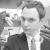## 易语言编程小神童教大家数组快速排序大小！

### 以下是问题补充：

@JSON.org.cn：我发明了一种语言, 叫J语言, 下面是: J语言编程小神童教大家数组快速排序大小！ loop( n in array) loop( n1 in array ) if( array[n] < array[n1] ) tmpSave = array[n] array[n]= array[n1]; array[n1]= tmpSave 呵呵简单吧？有什么不懂之处请大家尽情提问我！我会一一解答！ (2013/02/23 21:52)

0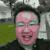0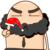#### 引用来自“流雨星_”的答案

```for(int i=0;i<n;i++){
for(int j=i+1;j<n;j++){
if(a[i]<a[j]){
int tmp = a[i];
a[i]=a[j];
a[j]=tmp;
}
}
}```
0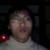0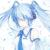0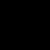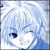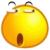0